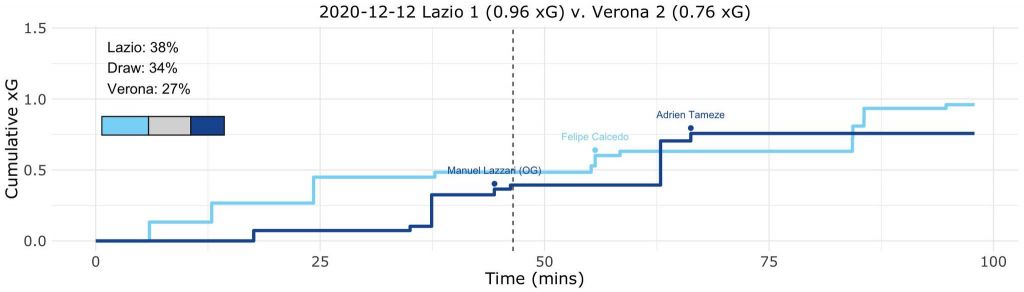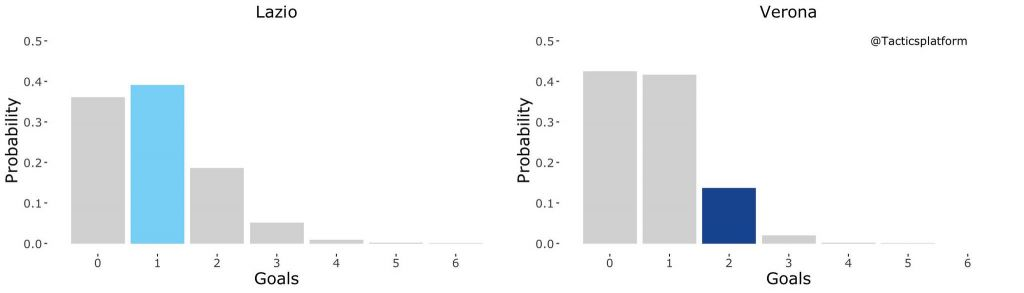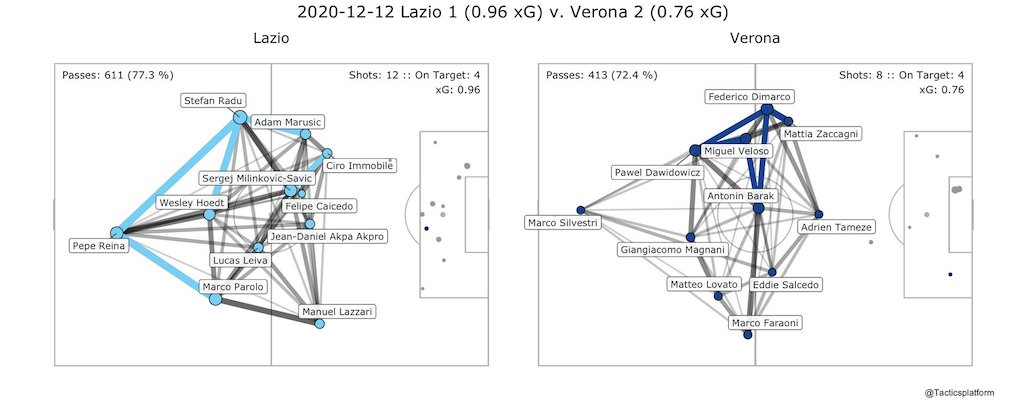In Matchday 11 of the 2020/21 Serie A, Lazio were defeated 2-1 by Hellas Verona at the Stadio Olimpico. Below is a statistical analysis of the game between the Biancocelesti and the Gialloblu.

##### Expected Goals (xG) Step PlotThe diagram above represents a summary of all simulations. The y-axis represents the cumulative xG (how many goals should be scored) and the x-axis represents time.

• Lazio: cumulative xG of 0.96
• Hellas Verona: cumulative xG of 0.76

In addition, the diagram above summarizes every shot taken by each club. Each shot is represented by a step and the height of the step measures the xG outcome of that shot; the higher the step, the more likely that the shot taken should have been converted. Furthermore, a dot with a name on the ‘Expected Goals Step Plot’ represents when a goal was scored and who the goal was scored by.

Finally, the colored bar in the top left of the diagram shows the probability of each club winning the match or a draw occurring.

• Lazio: 38%
• Hellas Verona: 27%
• Draw: 34%
##### Outcome Probability Bar ChartThe charts in the diagram above are a summary of a 10,000-time simulation of the probability of how many goals each side should have scored and the actual number of goals each side scored.

• Lazio: most likely to score 1 goal, actually scored 1 goal
• Hellas Verona: most likely to score 0 goals, actually scored 2 goals
##### Pass Network Plot & Shot Location PlotThe diagram above firstly shows the number of passes completed by each side in the match.

• Lazio: 611 passes with a 77.3% accuracy rate
• Hellas Verona: 413 passes with a 72.4% accuracy rate

Secondly, the diagram outlines the most active players for each team. Each player is represented by a node; the size of each node denotes the number of touches each player had.

Thirdly, the diagram indicates the pass connections between teammates. These connections are the shaded grey lines throughout the plots; the size and shade of the line between players indicates the frequency of a pass connection. The top five connections are outlined in light blue (Lazio) and blue (Hellas Verona); it is important to note that the direction of each connection is not shown.

Finally, this diagram shows the coordinates of each shot. The shots are plotted as light grey dots while goals are plotted as dark blue dots. The size of each dot represents the xG; the bigger the dot, the higher the chance that this opportunity should have found the back of the net (been a dark blue dot).

• Lazio: 12 total shots, 4 shots on target
• Hellas Verona: 8 total shots, 4 shots on target